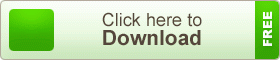# English Version HSC 1st Paper 6th Chapter Physics Note

English Version HSC 1st Paper 6th Chapter Physics Note. Gravitation and Gravity. The physics is one of the oldest academic disciplines, perhaps the oldest through the inclusion of astronomy. In the last two millennia, physics was a part of natural philosophy, chemistry, certain branches of mathematics and biology, but during the scientific revolution in the 17th century, the natural sciences emerged as unique in its own right research programs. Physics intersects with many interdisciplinary areas of research, such as Biophysics and quantum chemistry, and physics limits are not rigidly defined. New ideas in physics often explain the fundamentals of other sciences mechanisms, to open new avenues of research in areas such as mathematics and philosophy.## English Version HSC 1st Paper 6th Chapter Physics Note

Gravitation and Gravity

Question No.1

An object of 5kg mass is thrown from the earth’s surface by escape velocity to another planet of the space whose mass is 16 times of the earth and the diameter is 8 times of the earth radius (earth’s mass = 6 x 1024kg, earth’s radius = 6.4 X 103 km).

a. Write down the work-energy theorem. 1

b. The power of a machine is 50 MW-Explain. 2

c. Determine the value of gravitational acceleration on the surface of another planet as mentioned in the stem. 3

d. Will the required escape velocity of the body when its mass is half of the mass as mentioned in the stem is thrown from another planet be the same as the escape velocity at the earth’s surface? Give your opinion through mathematical analysis.

The answer to the question no. 1

a. The work done by the sum of all forces acting on a particle equals the change in the kinetic energy of the particle.

b. 50MW power of a machine means the machine can do 50MJ or 50 x 106J work per second or can convert 50MJ energy to another form of usable energies.

Let’s assume,

Mass of earth, = M

Given,

Mass of the other planet, M = 16M

The radius of the other planet, R’ = 4R

[: Diameter of that planet is 8 times earth’s radius]

g’=9.77ms-2

Question No.2a. What is angular acceleration? 1

b. Why is the velocity of a gas molecule zero at absolute temperature? Explain. 2

c. Determine the velocity of the satellite. 3

d. Which going from the planet to the satellite, will the resultant force on the space shuttle be zero at any place? Give your decision mathematically.

The answer to the question no. 2

a. When the time interval is infinitesimal, the rate of change in angular velocity is defined as the angular acceleration.

b. When gas molecules are kept in a container they move randomly in every direction of colliding with walls of the container. They also collide with each other. The number of collisions per second was estimated to be 109. Due to this continuous collisions, the velocity of gas molecules ranges from zero to infinity. And not only the magnitude of velocity change but also the direction. According to the kinetic theory of gases, the kinetic energy of one-mole gas, E = 3/2RT. At T = 0, E = 0, i.e., at absolute zero, there will be no kinetic energy, hence no velocity. Again rms velocity of molecules is proportional to square root of absolute temperature.Physics is one of the oldest academic disciplines and, through its inclusion of astronomy, perhaps the oldest. Over the last two millennia, physics, chemistry, biology, and certain branches of mathematics were a part of natural philosophy, but during the scientific revolution in the 17th century, these natural sciences emerged as unique research endeavors in their own right. Physics intersects with many interdisciplinary areas of research, such as biophysics and quantum chemistry, and the boundaries of physics are not rigidly defined. New ideas in physics often explain the fundamental mechanisms studied by other sciences and suggest new avenues of research in academic disciplines such as mathematics and philosophy.

teachingbd24.com is such a website where you will get all kinds of necessary information regarding educational notes, suggestions and question patterns of schools, colleges, and madrasas. Particularly, you will get here special notes of physics that will be immensely useful to both students and teachers. The builder of the website is Mr. Md. Shah Jamal who has been serving for 32 years as an Assistant Professor of Physics at BAF Shaheen College Dhaka. He expects that this website will meet up all the needs of Bengali version learners /students. He has requested concerned students and teachers to spread this website home and abroad.

## English Version HSC Physics Note